# Quiz 10: Production and Cost Estimation

Short run production functions shows the relationship between the variable factors and the quantity of output produced by employing variable factors of production. In short run some factors are fixed that is there amount can't be increased in short term. The data for short run production function is given below: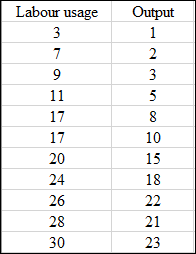a. To know whether a cubic function can be used as production function the scatter plot can be used. Using excel sheet draw the scatter plot from the category charts. The scatter for the given data of production function is given below: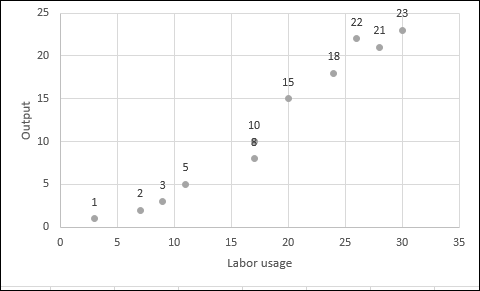X axis shows the Labor usage and Y axis of the graph shows the output level. The points shows the output for the corresponding value of labor usage. The scatter plot is an upward sloping curve with increasing slope. Therefore, a cubic production can be used to estimate the short run production function. b. To estimate the cubic function run a regression taking output as dependent variable and taking labor usage, square of labor usage and cube of labor usage as independent variable. The table showing the value of dependent and independent variables are given below: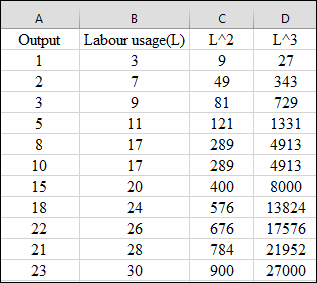Run the regression in excel by following these steps which are given below: 1. Go to options in file and click in Add-Ins and select analysis toolpak from there. 2. Select data from the toolbar and go to data analysis. 3. In data analysis go to regression. The dialogue box appear. 4. In the dialogue box put the value of dependent variable and in next column put the values of independent variables. 5. Then run the regression. The result for the regression will appear in other sheet of the Excel. The results are given below: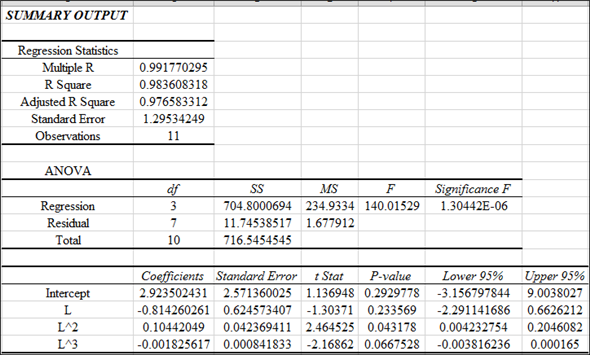So the equation for the value of dependent variable in terms of independent variables can be shown as given below: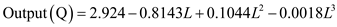The negative sign of coefficients of labor usage and cube of labor usage doesn't make sense because it means that with increase in labor usage the output will fall always which is not a possible case. Even these coefficients are not significant at 5% significance level as p value is smaller than 0.05. The coefficient of only square of labor usage is significant. c. The marginal product in the short run first increases then become constant and then starts falling. The marginal product is addition in output due to increase in variable input by one unit. To calculate the lowest value of labor for which marginal product will have a negative sign differentiate the production function with respect to L and equate it to zero. The differentiation of short run production function is given below: Marginal product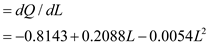The value of marginal product began to fall when the derivative of marginal product with respect to L will be negative. The derivative of marginal product is calculated below:The derivative of marginal product is equated to zero to find the value of L as shown below:So, after 19 units of labor usage, marginal product of labor will starts falling. d. The short run production function is given below:When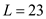the value of output can be calculated as shown below:The short run marginal product is given below: Marginal productWhenthe value of marginal product can be calculated as shown below:The average product is the quantity of output per unit of worker. The average product can be calculated by dividing the total output by total number of labor usage. The calculation for average product is shown below:e. To know whether short run MC is rising or falling when firms employ 23 labor units the value of marginal product should be known. If the value of marginal product at 23 labor units is positive that means marginal cost is falling due to increasing marginal product. The marginal product is 1.1315 at 23 units of labor. Therefore, the marginal cost must be decreasing at 23 units of labor usage.
The total product function is written as:The restrictions on parameters are: A 0 and B 0 - This is because for average and marginal products to first rise and then fall, A must be negative and B must be positive. - The marginal product becomes negative beyond the optimal level and starts giving diminishing returns. - Therefore A 0 and B 0 is inappropriate coefficient.
The following is the production function of D.F.C.: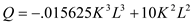(A) Given the value of K=8, the total product function would be:Average product function is derived by dividing L to total product:Marginal product (MP) function is derived by differentiating the total product function:(B) When the marginal product is equated zero, the number of labor units derived is maximum. After that unit, the marginal product starts falling:The marginal product starts becoming negative after 53 rd unit. (C) Substitute L=50 in TP, MP and AP:The total product is 600,000.The average product is 12000.The marginal product is 4000 at labor unit 50.